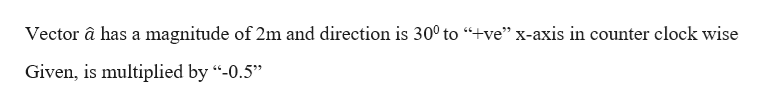# We start with vector a→of magnitude 2 m and direction 30° counterclockwise from the +x direction. If we multiply it by –0.5, what is the resulting vector’s angle (clockwise from the +x direction)?Thanks!

Question

We start with vector a→of magnitude 2 m and direction 30° counterclockwise from the +x direction. If we multiply it by –0.5, what is the resulting vector’s angle (clockwise from the +x direction)?

Thanks!

check_circleExpert Solution
Step 1

Given information:help_outlineImage TranscriptioncloseVector a has a magnitude of 2m and direction is 300 to "+ve" x-axis in counter clock wise Given, is multiplied by "-0.5" fullscreen
Step 2

When a vector is multiplied by a “-ve” integer its direction becomes completely opposite i.e. it turns an angle of 1800 and magnitude will be the multiple of the integer.

Hence the new vector makes an angle:

θ = 180 + 30 = 2100

Wit...

### Want to see the full answer?

See Solution

#### Want to see this answer and more?

Solutions are written by subject experts who are available 24/7. Questions are typically answered within 1 hour*

See Solution
*Response times may vary by subject and question
Tagged in

### Vectors and Scalars